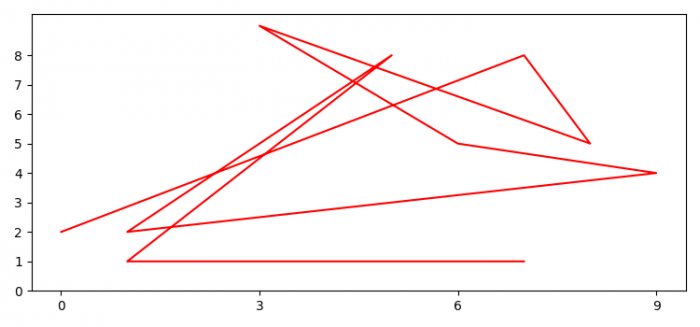# How to adjust 'tick frequency' in Matplotlib for string X-axis?

To adjust tick frequency for X-axis, we can take the following steps −

• Set the figure size and adjust the padding between and around the subplots.
• Initialize a variable, N, for number of sample data points.
• Create x and y data points using numpy.
• Plot x and y data points using plot() method.
• Initialize a variable freq_x to adjust the frequency of the xticks.
• Use xticks() method to set the xticks.
• To display the figure, use show() method.

## Example

import matplotlib.pyplot as plt
import numpy as np

plt.rcParams["figure.figsize"] = [7.50, 3.50]
plt.rcParams["figure.autolayout"] = True

N = 10

x = np.random.randint(low=0, high=N, size=N)
y = np.random.randint(low=0, high=N, size=N)

plt.plot(x, y, color='red')

freq_x = 3

plt.xticks(np.arange(0, N, freq_x))

plt.show()

## Output